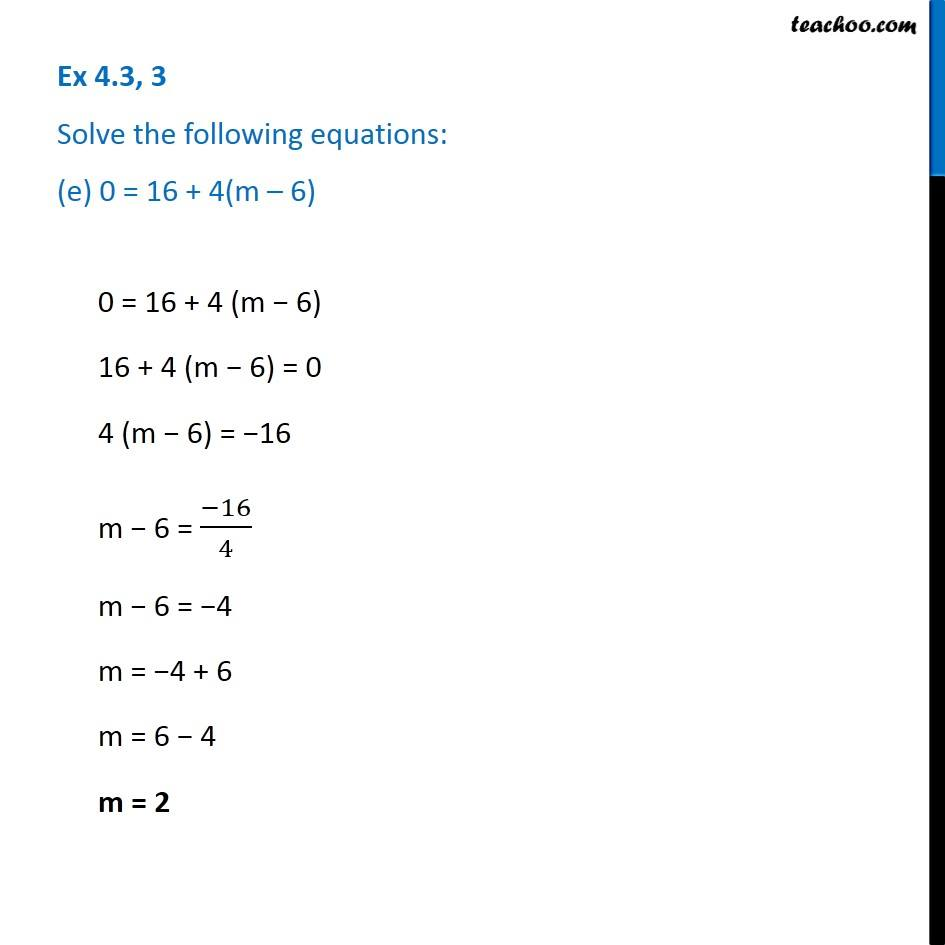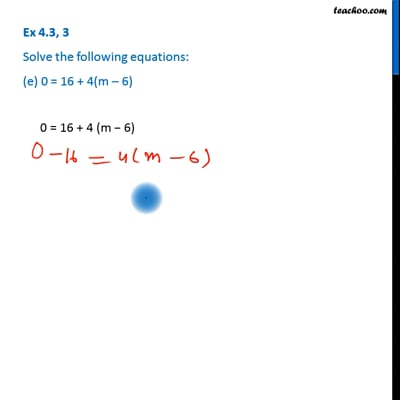Solving equations (with fractions)

Chapter 4 Class 7 Simple Equations
Serial order wiseThis video is only available for Teachoo black users

Learn in your speed, with individual attention - Teachoo Maths 1-on-1 Class

### Transcript

Question 3 Solve the following equations: (e) 0 = 16 + 4(m – 6) 0 = 16 + 4 (m − 6) 16 + 4 (m − 6) = 0 4 (m − 6) = −16 m − 6 = (−16)/4 m − 6 = −4 m = −4 + 6 m = 6 − 4 m = 2